JEE  >  BITSAT Physics Test - 8

# BITSAT Physics Test - 8

Test Description

## 40 Questions MCQ Test BITSAT Mock Tests Series & Past Year Papers | BITSAT Physics Test - 8

BITSAT Physics Test - 8 for JEE 2023 is part of BITSAT Mock Tests Series & Past Year Papers preparation. The BITSAT Physics Test - 8 questions and answers have been prepared according to the JEE exam syllabus.The BITSAT Physics Test - 8 MCQs are made for JEE 2023 Exam. Find important definitions, questions, notes, meanings, examples, exercises, MCQs and online tests for BITSAT Physics Test - 8 below.
Solutions of BITSAT Physics Test - 8 questions in English are available as part of our BITSAT Mock Tests Series & Past Year Papers for JEE & BITSAT Physics Test - 8 solutions in Hindi for BITSAT Mock Tests Series & Past Year Papers course. Download more important topics, notes, lectures and mock test series for JEE Exam by signing up for free. Attempt BITSAT Physics Test - 8 | 40 questions in 50 minutes | Mock test for JEE preparation | Free important questions MCQ to study BITSAT Mock Tests Series & Past Year Papers for JEE Exam | Download free PDF with solutions
 1 Crore+ students have signed up on EduRev. Have you?
BITSAT Physics Test - 8 - Question 1

### A capacitor of capacitance C has reactance X. If capacitance and frequency become double, then the capacitive reactance will be

Detailed Solution for BITSAT Physics Test - 8 - Question 1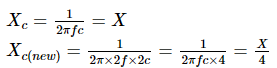BITSAT Physics Test - 8 - Question 2

### Two deutrons each of mass m fuse to form helium resulting in release of energy E. The mass of helium formed is

BITSAT Physics Test - 8 - Question 3

### Fusion reaction is initiated with the help of

BITSAT Physics Test - 8 - Question 4

A cell of emf 6 V and resistance 0.5 ohm is short circuited. The current in the cell is

Detailed Solution for BITSAT Physics Test - 8 - Question 4  i = E/r = 6/0.5 = 12amp.
BITSAT Physics Test - 8 - Question 5

A galvanometer of resistance 36 Ω is changed into an ammeter by using a shunt of 4 Ω. The fraction f₀ of total current passing through the galvanometer is

BITSAT Physics Test - 8 - Question 6

An electron of mass m when accelerated through a potential difference V has de Broglie wavelength λ. The de Broglie wavelength associated with a proton of mass M accelerated through the same potential difference will be

BITSAT Physics Test - 8 - Question 7

The mass of a particle is 400 times than that of an electron and the charge is double. The particle is accelerated by 5 V. Initially the particle remained in rest, then its final kinetic energy will be

BITSAT Physics Test - 8 - Question 8

In an L-R circuit, the value of L is (0.4/π) henry and the value of R is 30 ohm. If in the circuit, an alternating emf of 200 volt at 50 cycles per second is connected, the impedance of the circuit and current will be

Detailed Solution for BITSAT Physics Test - 8 - Question 8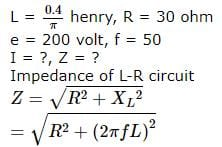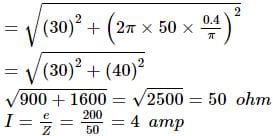BITSAT Physics Test - 8 - Question 9

The capacitor of a parallel plate capacitor with no dielectric substance and a separation of 0.4 cm is 2 μF. The separation is reduced to half and it is filled with a substance of dielectric value 2.8. The new capacity of the capacitor is

BITSAT Physics Test - 8 - Question 10

An electric field is given by Ex = −x3 kN/C . The potential of the point (1, -2) if the potential of the point (2, 4) is taken as zero, is

BITSAT Physics Test - 8 - Question 11

A motorcyclist of mass m is to negotiate a curve of radius r with a speed ν. The minimum value of the coefficient of friction so that this negotiation may take place safely, is

BITSAT Physics Test - 8 - Question 12

A clock S is based on oscillation of a spring and a clock P is based on pendulum motion. Both clocks run at the same rate on earth. On a planet having the same density as earth but twice the radius

BITSAT Physics Test - 8 - Question 13

At a common temperture, a block of wood and a block of metal feel equally cold or hot. The temperatures of block of wood and block of metal are

BITSAT Physics Test - 8 - Question 14

A gas at 300 K, enclosed in a container is placed in a fast moving train. When the train is in motion, then temperature of the gas

BITSAT Physics Test - 8 - Question 15

A particle of mass M and charge Q moving with velocity ν̅ describes a circular path of radius R when subjected toa uniform transverse magnetic field of induction B. the work done by the field when the particle completes one full circle is

BITSAT Physics Test - 8 - Question 16

A bar magnet when placed at an angle of 30o to the direction of magnetic field induction of 5*10− 5 T , experiences a moment of couple 2.5*10− 6 N-m. If the length of the magnet is 5cm its pole strength is:

BITSAT Physics Test - 8 - Question 17

If iron pieces loaded in a ship, are thrown into the water, then level of water will

BITSAT Physics Test - 8 - Question 18

A spherical ball of radius r is falling in a viscous fluid of viscosity h with a velocity v. The retarding viscous force acting on the spherical ball is

BITSAT Physics Test - 8 - Question 19

Two wires of same radius and length are subjected to the same load. One wire is of steel and the other is of copper. If Young's modulus of steel is twice that of copper, then ratio of elastic potential energies stored per unit volume in steel wire to that in copper wire is

BITSAT Physics Test - 8 - Question 20

Tom and Dick are running forward with the same speed. They are throwing a rubber ball to each other at a constant speed V as seen by the thrower. According to Sam who is standing on the ground the speed of ball is

BITSAT Physics Test - 8 - Question 21

A stone is tied to one end of a spring 50 cm long is whirled in a horizontal circle with a constant speed. If the stone makes 10 revolutions in 20 s, what is the magnitude of acceleration of the stone

BITSAT Physics Test - 8 - Question 22

A stone of mass m is tied to a string and is moved in a vertical circle of radius r making n revolutions per minute. The total tension in the string when the stone is at its lowest point is

BITSAT Physics Test - 8 - Question 23

A cold soft drink is kept on the balance. When the cap is open, then the weight

BITSAT Physics Test - 8 - Question 24

The ability of eye to focus near as well as distant objects is called

BITSAT Physics Test - 8 - Question 25

A particle, with restoring force proportional to displacement and resisting force proportional to velocity is subjected to a force F sin ωt. If the amplitude of the particle is maximum for ω=ω₁ and the energy of the particle is maximum for ω=ω₂ then

BITSAT Physics Test - 8 - Question 26

A simple pendulum of length 'l' has a maximum angular displacement (θ). The energy of a bob of mass m at the end of the displacement is

BITSAT Physics Test - 8 - Question 27

The angle of minimum deviation for an incident light ray on an equilateral prism is equal to its refracting angle. The refractive index of its material is

BITSAT Physics Test - 8 - Question 28

A solid sphere is moving on a horizontal plane. Ratio of its translational K.E. and rotational energy is

BITSAT Physics Test - 8 - Question 29

An inclined plane makes an angle of 30o with the horizontal. A solid sphere rolling down this inclined plane from rest without slipping has a linear acceleration equal to

BITSAT Physics Test - 8 - Question 30

Consider the following two statements A and B, and identify the correct choice of given answers. (A) Thermo e.m.f is minimum at neutral temperature of a thermocouple (B)When two junctions made of two different metallic wires are maintained at different temperatures, an electric current is generated in the circuit

BITSAT Physics Test - 8 - Question 31

A particle performs uniform circular motion with an angular momentum L. If the frequency of particle's motion is doubled and its kinetic energy is halved, the angular momentum becomes

BITSAT Physics Test - 8 - Question 32

Which of the following can not determine the state of a thermodynamic system

BITSAT Physics Test - 8 - Question 33

A monoatomic gas (γ = 5/3 ) is suddenly compressed to 1/8 of its original volume adiabatically, then the pressure of the gas will change to

Detailed Solution for BITSAT Physics Test - 8 - Question 33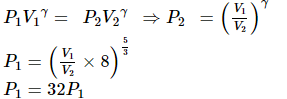BITSAT Physics Test - 8 - Question 34

In Huygen's wave theory, the locus of all points oscillating in the same phase is called a

BITSAT Physics Test - 8 - Question 35

If m is mass, Q is charge and B is magnetic induction, m/BQ has the same dimensions as

Detailed Solution for BITSAT Physics Test - 8 - Question 35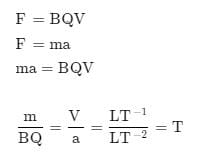T = 1/F

BITSAT Physics Test - 8 - Question 36

For the constructive interference the path difference between the two interfering waves must be equal to

BITSAT Physics Test - 8 - Question 37

A string of 7 m length has a mass of 0.035 kg. If tension in the string is 60.5 N, then velocity of a wave in the string is

BITSAT Physics Test - 8 - Question 38

A sonometer wire is vibrating in the second overtone. It means that there are

BITSAT Physics Test - 8 - Question 39

A ball moving with speed ν hits another identical ball at rest, The two balls stick together after collision. If specific heat of the material of the balls is S, the temperature rise resulting from the collision is

BITSAT Physics Test - 8 - Question 40

A 4 kg mass and a 1 kg mass are moving with equal kinetic energies. The ratio of the magnitudes of their linear momenta is

## BITSAT Mock Tests Series & Past Year Papers

2 videos|15 docs|70 tests
 Use Code STAYHOME200 and get INR 200 additional OFF Use Coupon Code
Information about BITSAT Physics Test - 8 Page
In this test you can find the Exam questions for BITSAT Physics Test - 8 solved & explained in the simplest way possible. Besides giving Questions and answers for BITSAT Physics Test - 8, EduRev gives you an ample number of Online tests for practice

## BITSAT Mock Tests Series & Past Year Papers

2 videos|15 docs|70 tests